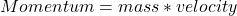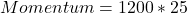Question

2. Which of the following objects has the greatest momentum?
A) a 145 grain baseball hit at 45 m/s
B) a 1200 kg car driving 25 m/s
C) a 2.0 *10-2 kg snail moving al 3.0 * 104 m/s
D) a 500 kg horse galloping at 12.0 m/s

1.niczorrrr

B) a 1200 kg car driving 25 m/s

Explanation:

Momentum can be defined as the multiplication (product) of the mass possessed by an object and its velocity. Momentum is considered to be a vector quantity because it has both magnitude and direction.

Mathematically, momentum is given by the formula;This ultimately implies that, the mass of an object or body is directly proportional to its momentum. Thus, the higher the mass of an object or body, the greater would be its momentum and vice-versa.

By mere inspection of the data given, we can see that the object with the greatest amount of mass and velocity is the car weighing 1200 kilograms and moving at 25 meters per seconds.

Substituting into the formula, we have;Momentum = 30,000 Kgm/s